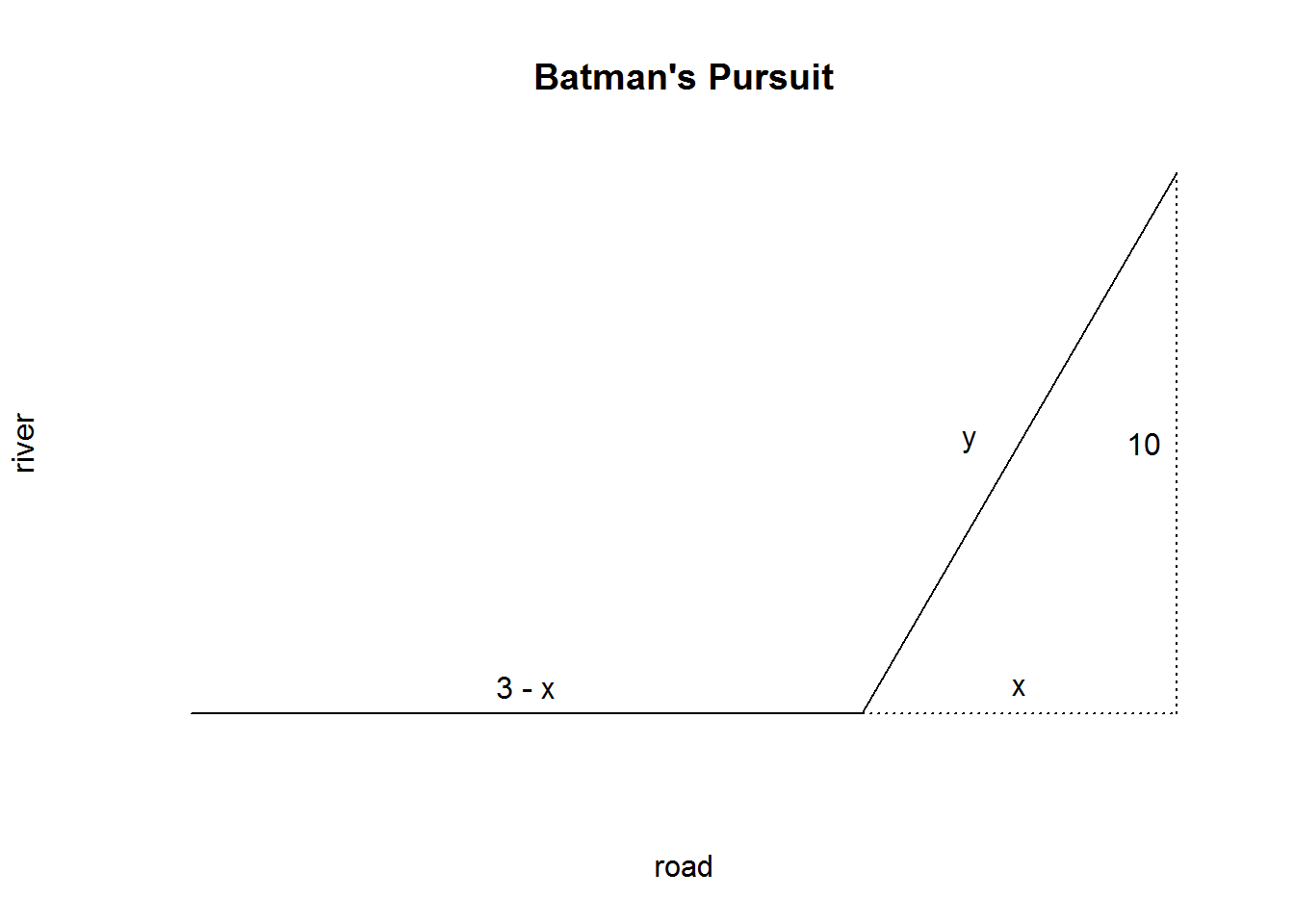Batman receives a distress beacon: Poison Ivy and her crew are attacking the D’Angelo sewage treatment plant. Batman is already in the Batmobile, but unfortunately, the bridges are blocked due to rush-hour traffic. He has to drive around the outskirts. Knowing that he can drive 5 times faster than the can glide, he quickly does the algebra and computes that the objective is to minimize the time $T(x,y) = 3 - x + 5y$ but he cannot be bothered to do the calculations while in pursuit of a criminal. Find the critical point $$x$$ for where Batman should eject from the Batmobile and then use his Bat-Glider over Gotham River to get a drop on Ivy.

x <- seq(0,3,0.1)
y <- x/3
plot(x,y, frame.plot = F, main = "Batman's Pursuit", pch = " ",
xaxt = "n", xlab = "road", yaxt = "n", ylab = "river")

lines( c(0,sqrt(100/24)), c(0,0) )
lines( c(sqrt(100/24),3), c(0,1) )
lines( c(sqrt(100/24),3), c(0,0), lty = 3 )
lines( c(3,3), c(0,1), lty = 3 )

text( 0.5*(sqrt(100/24)), 0.05, "3 - x")
text( 0.5*(sqrt(100/24) + 3), 0.05, "x")
text( 0.47*(sqrt(100/24) + 3), 0.1*(sqrt(100/24) + 3), "y")
text( 2.9, 0.5, "10")